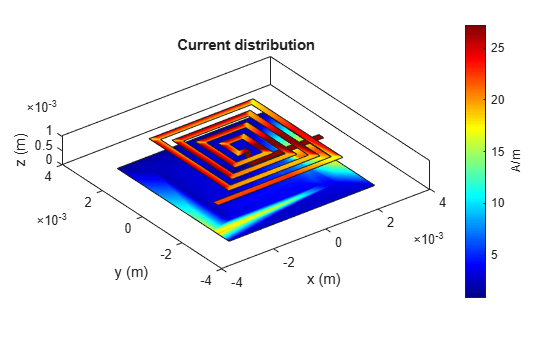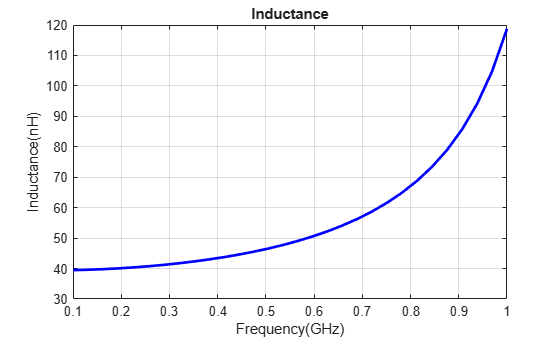# Spiral Inductor - Visualize and Analyze

This example shows you how to create, visualize, and analyze a spiral inductor.

Create a spiral inductor with default properties.

`inductor = spiralInductor`
```inductor = spiralInductor with properties: SpiralShape: 'Square' InnerDiameter: 5.0000e-04 Width: 2.5000e-04 Spacing: 2.5000e-04 NumTurns: 4 Height: 0.0010 GroundPlaneLength: 0.0056 GroundPlaneWidth: 0.0056 Substrate: [1×1 dielectric] Conductor: [1×1 metal] ```

View the inductor.

`show(inductor)`Plot the charge distribution at 500 MHz.

`charge(inductor, 500e6)`Plot the current distribution at 500 MHz.

```figure current(inductor, 500e6)```Calculate and plot the inductance.

```figure; inductance(inductor,linspace(100e6,1e9,30));```